• Save

# Compound Interest Questions and Answers for Banks Exam and SSC

5 months ago 5.7K Views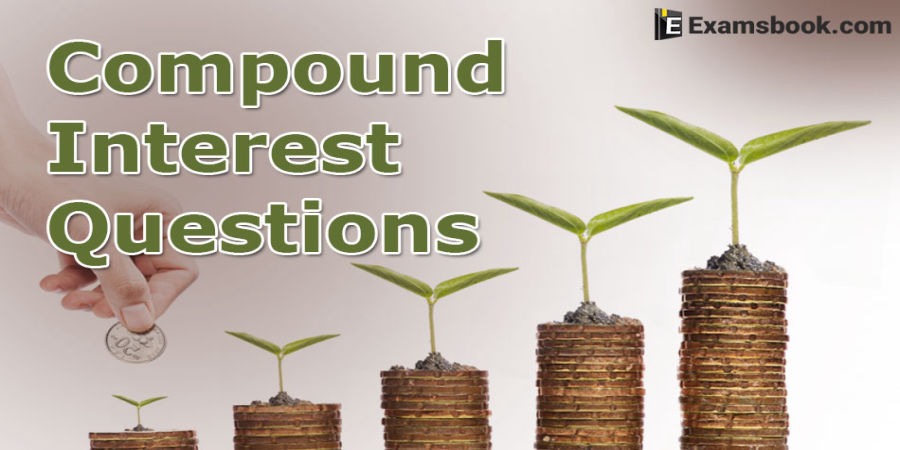Here are the selective and important Compound interest questions and answers for banks exam and SSC. These Compound interest questions have been asked in competitive exams and there are chances to ask again in other competitive exams.

Practice with Compound interest questions and answers for better results in exams. Try to solve Compound interest questions yourself with the help of answers and check your performance. All the best.

## Compound interest questions and answers for competitive exams

1. What is the difference between the compound interests on Rs. 5000 for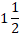years at 4% per annum compounded yearly and half-yearly?

(A) Rs. 2.04

(B) Rs. 3.06

(C) Rs. 4.80

(D) Rs. 8.30

(E) none of these

Ans .  A

2.The principle that amounts to Rs. 4913 in 3 years at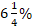per annum compound interest compounded annually, is:

(A) Rs. 3096

(B) Rs. 4076

(C) Rs. 4085

(D) Rs. 4096

Ans .  D

3. If the compound interest on a sum for 2 year at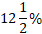per annum is Rs. 510, the simple interest on the same sum at the same rate for the same period of time is

(A) Rs.400

(B) Rs.450

(C) Rs.460

(D) Rs.480

Ans .  D

4. The simple interest on a certain sum of money for 3 year at 8% per annum is half the compounded interest on Rs. 4000 for 2 years at 10% per annum. The sum placed on simple interest is:

(A) Rs.1550

(B) Rs. 1650

(C) Rs. 1750

(D) Rs. 2000

Ans .  C

5. The difference between simple and compound interests compounded annually on a certain sum of money for 2 years at 4 % per annum is Rs. 1. The sum (in Rs.) is:

(A) 625

(B) 630

(C) 640

(D) 650

Ans .  A

6. The effective annual rate of interest corresponding to a nominal rate of 6% per annum payable half-yearly is:

(A) 6.06%

(B) 6.07%

(C) 6.08%

(D) 6.09%

Ans .  D

7. A sum of money invested at compound interest amounts to Rs. 800 in 3 years and to Rs.840 in 4 years. The rate of interest per annum is:

(A)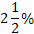(B) 4%

(C) 5%

(D)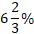Ans .  C

8. What annual payment will discharge a debt of Rs. 1025 due in 2 years at the rate of 5% compound interest?

(A) Rs. 550

(B) Rs. 551.25

(C) Rs. 560

(D) Rs. 560.75

Ans .  B

If you face any problems in compound interest questions and answers, feel free and ask me in the comment section. Visit on the next page for more practice

## About author

### Vikram Singh

Providing knowledgable questions of Reasoning and Aptitude for the competitive exams.

## Report Error : Compound Interest Questions and Answers for Banks Exam and SSC

Please Enter Message
Error Reported Successfully